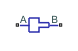# Area Change (IL)

Area expansion or contraction along a pipe in an isothermal liquid network

• Library:
• Simscape / Fluids / Isothermal Liquid / Pipes & Fittings

•## Description

The Area Change (IL) block models a sudden or a gradual area change along a pipe with fixed areas and variable flow direction. When the fluid moves from port A to port B, it experiences an area contraction. When the fluid flows from port B to port A, it experiences an area expansion. The inlet and outlet areas can be equal.

Both semi-empirical and tabular formulations are available for correlating flows to losses.

### Semi-Empirical Formulation

In the semi-empirical, analytical formulation, losses in pressure and velocity are characterized by a Hydraulic loss coefficient, K, in terms of a user-defined Contraction correction factor, Ccontraction, and Expansion correction factor, Cexpansion, from Crane . The coefficient that characterizes area change is calculated from both expansion and contraction loss factors and based on the flow rate through the block.

For gradual conical area contractions between 0 and 45 degrees, the contraction loss factor is:

`${K}_{contraction}=0.8{C}_{contraction}\mathrm{sin}\left(\frac{\theta }{2}\right)\left(1-R\right),$`

where R is the port area ratio $\frac{{A}_{smaller}}{{A}_{bigger}}.$ For gradual area contraction between 45 and 180 degrees:

`${K}_{contraction}=\frac{{C}_{contraction}}{2}\sqrt{\mathrm{sin}\left(\frac{\theta }{2}\right)}\left(1-R\right),$`

where θ is the Cone angle. A sudden area change has an angle of 180 degrees. In this case, the loss factor is calculated as ${K}_{contraction}=\frac{C}{2}\left(1-R\right).$

For gradual conical area expansions between 0 and 45 degrees, the expansion loss factor is:

`${K}_{expansion}=2.6{C}_{expansion}\mathrm{sin}\left(\frac{\theta }{2}\right){\left(1-R\right)}^{2},$`

and for gradual area expansion between 45 and 180 degrees:

`${K}_{expansion}={C}_{expansion}{\left(1-R\right)}^{2}.$`

The hydraulic loss coefficient for the pipe change segment is calculated from these values as:

`$K={K}_{expansion}+\frac{{K}_{contraction}-{K}_{expansion}}{2}\left(\mathrm{tanh}\left(\frac{3{\stackrel{˙}{m}}_{A}}{{\stackrel{˙}{m}}_{th}}\right)+1\right),$`

where:

• $\stackrel{˙}{m}$A is the mass flow rate through port A. Mass is conserved through the segment: ${\stackrel{˙}{m}}_{A}+{\stackrel{˙}{m}}_{B}=0.$

• ${\stackrel{˙}{m}}_{th}$ is the threshold mass flow rate for flow reversal, which is based on the Critical Reynolds number, Rec:

`${\stackrel{˙}{m}}_{th}=\frac{{\mathrm{Re}}_{c}{A}_{R}\upsilon \overline{\rho }}{{D}_{h}},$`

where:

• AR is the smallest segment area (either the Cross-sectional area at port A or the Cross-sectional area at port B.)

• ν is the fluid kinematic viscosity.

• $\overline{\rho }$ is the average fluid density.

• Dh is the hydraulic diameter at AR: ${D}_{h}=\sqrt{\frac{4{A}_{R}}{\pi }}.$

### Tabulated Data Parameterization

The loss factor can also be parameterized with user-provided data interpolated from the Reynolds number at the smallest area, which in turn is a function of the Critical Reynolds number:

`$K=TLU\left({\mathrm{Re}}_{c}\right).$`

Linear interpolation is employed between data points, and nearest-neighbor extrapolation is employed beyond the table boundaries.

### Pressure Differential

The pressure differential over the area change is

`${p}_{A}-{p}_{B}=\frac{{\stackrel{˙}{m}}^{2}}{2\overline{\rho }{A}_{R}^{2}}\left(1-{R}^{2}\right)+\Delta {p}_{loss},$`

where the pressure loss is:

`$\Delta {p}_{loss}=\frac{K}{2\overline{\rho }{A}_{R}^{2}}{\stackrel{˙}{m}}_{A}\sqrt{{\stackrel{˙}{m}}_{A}^{2}+{\stackrel{˙}{m}}_{th}^{2}}.$`

## Ports

### Conserving

expand all

Liquid entry port.

Liquid exit port.

## Parameters

expand all

Angle of expansion for a gradual area change parameterized as a cone.

#### Dependencies

To enable this parameter, set Local loss parameterization to ```Semi-empirical correlation - gradual area change```.

Model of hydraulic losses due to area change. You can choose from two analytical, semi-empirical formulations or you can provide your own data by selecting ```Tabulated data - loss coefficient vs. Reynolds number```.

Area at the section entrance, port A.

Area at the section exit, port B.

Vector of Reynolds number values for the tabular parameterization of the area change. The elements must correspond one-to-one with the elements of the Contraction loss coefficient vector and the Expansion loss coefficient vector parameters. The vector values are listed in ascending order.

#### Dependencies

To enable this parameter, set Local loss parameterization to ```Tabulated data - loss coefficient vs. Reynolds number```.

Vector of loss coefficients for an area contraction corresponding to the Reynolds number vector parameter, where n is the length of the Reynolds number vector. The elements are listed in descending order and must be greater than 0.

#### Dependencies

To enable this parameter, set Local loss parameterization to ```Tabulated data - loss coefficient vs. Reynolds number```.

Vector of loss coefficients for an area expansion corresponding to the Reynolds number vector parameter, where n is the length of the Reynolds number vector. The elements are listed in descending order and must be greater than 0.

#### Dependencies

To enable this parameter, set Local loss parameterization to ```Tabulated data - loss coefficient vs. Reynolds number```.

Coefficient used in the semi-empirical calculation of the area expansion loss factor.

#### Dependencies

To enable this parameter, set Local loss parameterization to either:

• ```Semi-empirical correlation - sudden area change```

• ```Semi-empirical correlation - gradual area change```

Coefficient used in the semi-empirical calculation of the area contraction loss factor.

#### Dependencies

To enable this parameter, set Local loss parameterization to either:

• ```Semi-empirical correlation - sudden area change```

• ```Semi-empirical correlation - gradual area change```

Upper Reynolds number limit for laminar flow through the orifice.

 Flow of Fluids Through Valves, Fittings, and Pipe, Crane Valves North America, Technical Paper No. 410M

 Idelchik, I.E., Handbook of Hydraulic Resistance, CRC Begell House, 1994